5 Operations on Lists
There are thousands of functions in the Wolfram Language that work with lists.
 In:=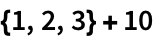Out=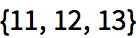In:=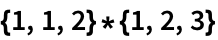Out=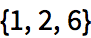Compute the first 10 squares:
 In:=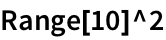Out=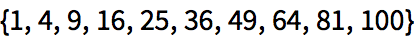Plot the first 20 squares:
 In:=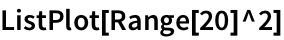Out=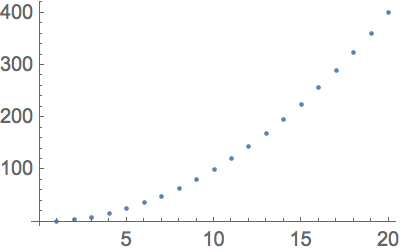Sort sorts a list into order:
 In:=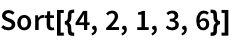Out=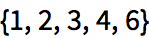Length finds how long a list is:
 In:=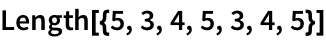Out=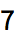Total gives the total from adding up a list:
 In:=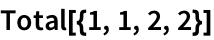Out=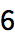Find the total of the numbers from 1 to 10:
 In:=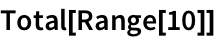Out=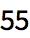Count counts the number of times something appears in a list.
Count the number of times a appears in the list:
 In:=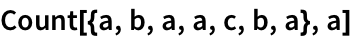Out=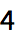Its often useful to be able to get individual elements of a list. First gives the first element; Last gives the last element. Part gives the element at a particular position.
Pick out the first element of a list:
 In:=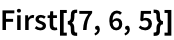Out=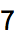Pick out the last element:
 In:=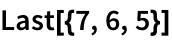Out=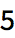Pick out element number 2:
 In:=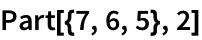Out=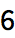Picking out the first element in a list youve sorted is the same as finding the minimum element:
 In:=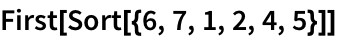Out=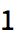In:=Out=Break a number into a list of digits:
 In:=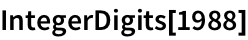Out=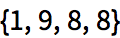Find the last digit:
 In:=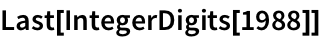Out=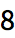Take lets you take a specified number of elements from the beginning of a list.
Take the first 3 elements from a list:
 In:=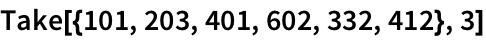Out=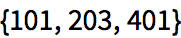Take the first 10 digits of 2 to the power 100:
 In:=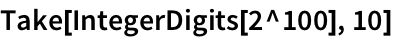Out=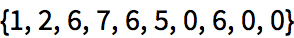Drop drops elements from the beginning of a list.
 In:=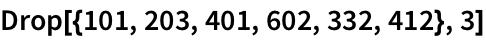Out=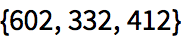{2,3,4}+{5,6,2} arithmetic on lists Sort[{5,7,1}] sort a list into order Length[{3,3}] length of a list (number of elements) Total[{1,1,2}] total of all elements in a list Count[{3,2,3},3] count occurrences of an element First[{2,3}] first element in a list Last[{6,7,8}] last element in a list Part[{3,1,4},2] particular part of a list, also written as {3, 1, 4}[] Take[{6,4,3,1},2] take elements from the beginning of a list Drop[{6,4,3,1},2] drop elements from the beginning of a list IntegerDigits list of digits in a number
5.1Make a list of the first 10 squares, in reverse order. »
Expected output:
 Out[]=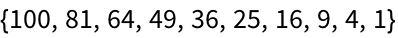Answer & check your solution
5.2Find the total of the first 10 squares. »
Expected output:
 Out[]=Answer & check your solution
5.3Make a plot of the first 10 squares, starting at 1. »
Expected output:
 Out[]=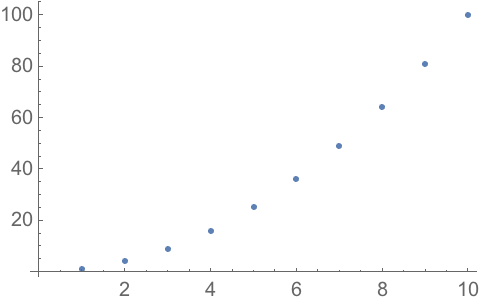Answer & check your solution
5.4Use Sort, Join and Range to create {1, 1, 2, 2, 3, 3, 4, 4}»
Expected output:
 Out[]=Answer & check your solution
5.5Use Range and + to make a list of numbers from 10 to 20, inclusive. »
Expected output:
 Out[]=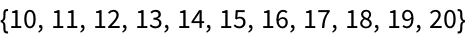Answer & check your solution
Expected output:
 Out[]=Answer & check your solution
5.7Find the number of digits in 2^128. »
Expected output:
 Out[]=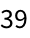Answer & check your solution
5.8Find the first digit of 2^32. »
Expected output:
 Out[]=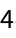Answer & check your solution
5.9Find the first 10 digits in 2^100. »
Expected output:
 Out[]=Answer & check your solution
5.10Find the largest digit that appears in 2^20. »
Expected output:
 Out[]=Answer & check your solution
5.11Find how many zeros appear in the digits of 2^1000. »
Expected output:
 Out[]=Answer & check your solution
5.12Use Part, Sort and IntegerDigits to find the second-smallest digit in 2^20. »
Expected output:
 Out[]=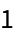Answer & check your solution
5.13Make a line plot of the sequence of digits that appear in 2^128. »
Expected output:
 Out[]=Answer & check your solution
5.14Use Take and Drop to get the sequence 11 through 20 from Range»
Expected output:
 Out[]=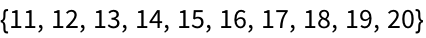Answer & check your solution
+5.1Make a list of the first 10 multiples of 3. »
Expected output:
 Out[]=Answer & check your solution
+5.2Make a list of the first 10 squares using only Range and Times»
Expected output:
 Out[]=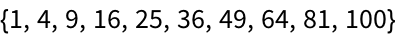Answer & check your solution
+5.3Find the last digit of 2^37. »
Expected output:
 Out[]=Answer & check your solution
+5.4Find the second-to-last digit of 2^32. »
Expected output:
 Out[]=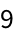Answer & check your solution
+5.5Find the sum of all the digits of 3^126. »
Expected output:
 Out[]=Answer & check your solution
+5.6Make a pie chart of the sequence of digits that appear in 2^32. »
Expected output:
 Out[]=Answer & check your solution
+5.7Make a list of pie charts for the sequence of digits in 2^20, 2^40, 2^60. »
Expected output:
 Out[]=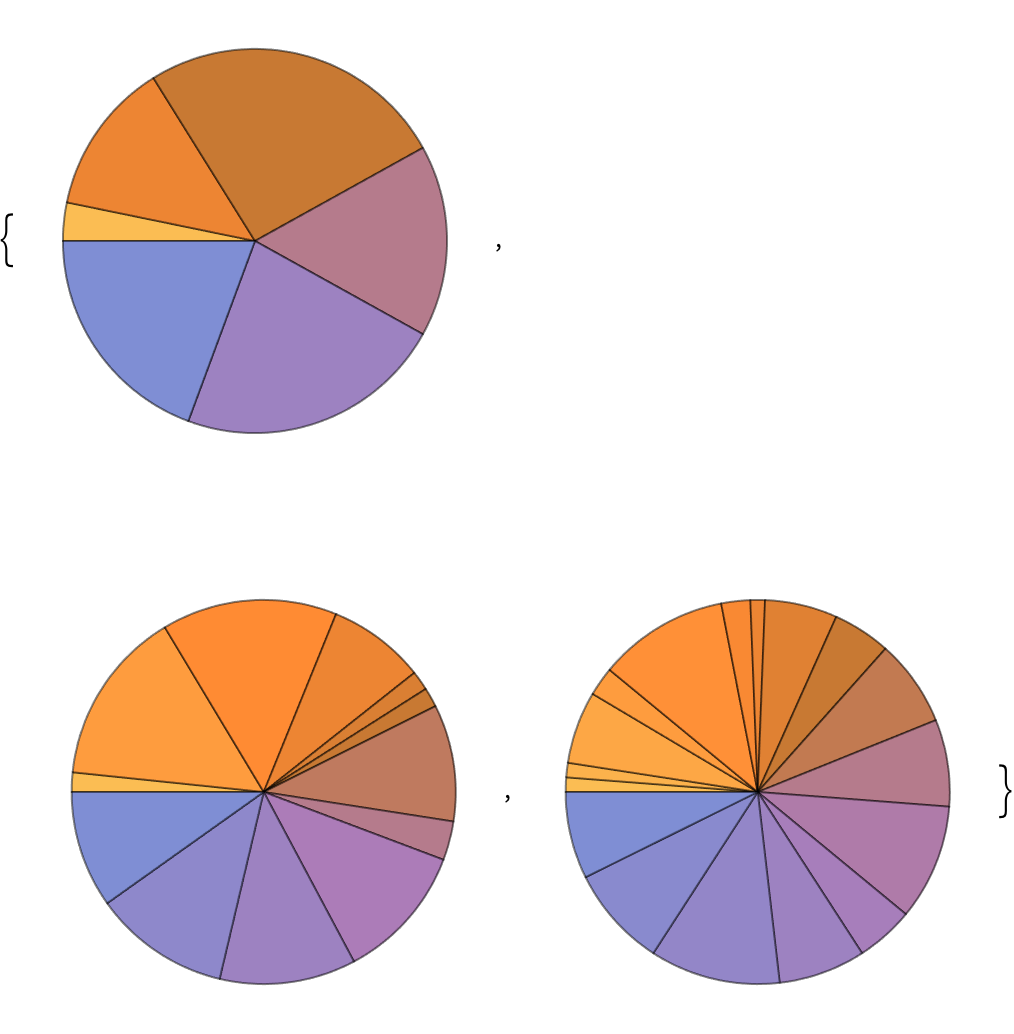Answer & check your solution
Can one add lists of different lengths?
No. {1, 2}+{1, 2, 3} wont work. {1, 2, 0}+{1, 2, 3} would be fine, if thats what you mean.
Yes. {} is a list of length 0, with no elements. Its usually called the null list or the empty list.
• IntegerDigits gives digits in base 10. IntegerDigits[5671, 2] gives digits in base 2. You can use any base you want. FromDigits[{5, 6, 7, 1}] reconstructs a number from its list of digits.
• Rest[list] gives all the elements of list after the first one. Most[list] gives all elements other than the last one.